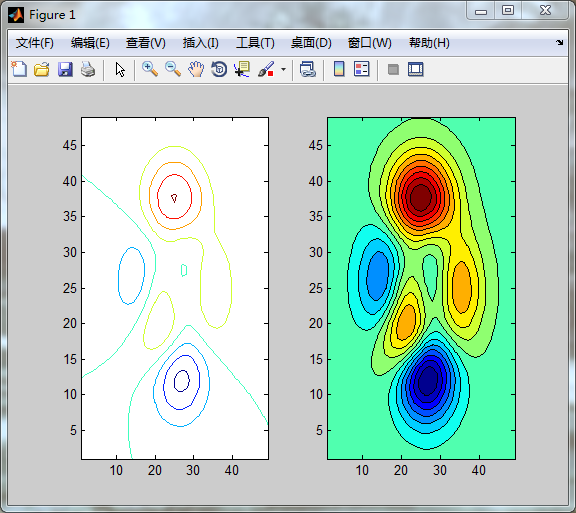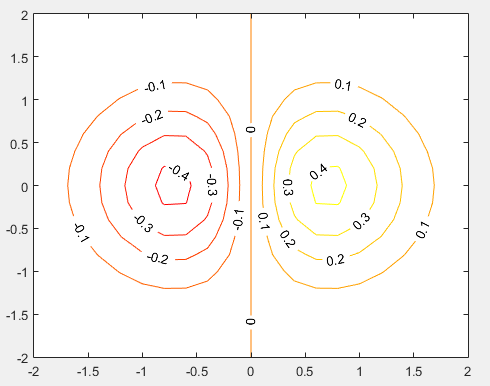• ## matlab绘制等高线图

万次阅读 多人点赞 2018-04-13 17:40:53
MATLAB提供函数contour（）绘制等高线图，函数contourf（）绘制经过填充的等高线图，具体调用格式如下：★ contour（z）：该函数绘制矩阵z的等高线。★ contour（x，y，z）：该函数在指定坐标（x，y）下，出矩阵 z...
MATLAB提供函数contour（）绘制等高线图，函数contourf（）绘制经过填充的等高线图，具体调用格式如下：
★ contour（z）：该函数绘制矩阵z的等高线。
★ contour（x，y，z）：该函数在指定坐标（x，y）下，画出矩阵 z 的等高线。
★ contour（z，n）：该函数绘制n条等高线。
★ contour（x，y，z，[v v]）：该函数绘制高度为 v 的等高线。
>> [x y z]=peaks;>> figure(1);>> subplot(121);>> contour(z);  %绘制函数的等高线>> subplot(122);
>> contourf(z,16);  %绘制等高线指定条数16并填充颜色------风雨无阻，勇往直前------
展开全文contour
• 您是否曾经想在极坐标中绘制数据的等高线图，但又不想经历将其全部转换为笛卡尔坐标的麻烦？ 好吧，如果是这样，这就是你的答案。 一个简单的 scriptlet，其工作方式与 MATLAB 的内置等高线绘图功能完全相同。 您...
• ## matlab绘制等高线图

万次阅读 2018-02-08 15:51:30
如何用散点绘制等高线图通过已知的山地海拔数据，利用MATLAB软件使用插值法绘制山地曲面图、山地等高线图、伪彩色图、散点图。工具/原料电脑MATLAB软件方法/步骤1将已知的海拔数据放入一个矩阵A中...
已知A=[X,Y,Z]数组，B=[X,Y,F]数组，想要数组A以X,Y,为坐标，Z为值画等高线图。再画
展开全文• % 等高线矩阵的获取 C=contourc(peaks(20),3);...% 等高线图形的绘制 contour(peaks(20),10);colormapautumn % 绘制二维的等高线 contour3(peaks(20),10);colormapautumn% 绘制三维的等高线 ...


% 等高线矩阵的获取

C = contourc(peaks(20),3);              % 获取3个等级的等高线矩阵

% 等高线图形的绘制

contour(peaks(20),10);colormap
autumn
% 绘制二维的等高线

contour3
(peaks(20),10);colormap
autumn

% 绘制三维的等高线

% 还可以指定等高线的条数、坐标系的比例及某高度上的等高线

% 等高线图形的填充

contourf(peaks(20),10);colormap
autumn
%绘制填充的二维等高线,有n的等级

%等高线图形的标注

[x,y]=meshgrid(-2:.2:2);
%生成格网

z=x.^exp(-x.^2-y.^2);   %函数

[C,h]=contour(x,y,z);colormap autumn;

%-----方法一

text_handle = clabel(C,h);

set(text_handle,'BackgroundColor',[1 1 .6],'Edgecolor',[.7 .7 .7])

%标注颜色设置为黄色           %标注的“边界”设置为灰色

%-----方法二

clabel(C,h,'FontSize',10,'Color','r','LabelSpacing',72,'Rotation',0);

%字体大小         %颜色      %两个标注的间隔    %标注（字）是否旋转

Contour Plots等高线矩阵的数据结构

[C,h]=contour(x,y,z);colormap
autumn
;

% C是等高线矩阵，包括x,y坐标和该点等高线的等级

% C的记录格式如下图

height1x1……xN1height2……点数N1y1……yN1点数N2……

注1：height1和height2可能相等，因为一个高度可能有多条等高线

注2：当等高线被边界截断时，等高线是不闭合的；闭合的等高线第一个点与最后一个点相等。

注3：h.ContourMatrix同样可以获取该等高线矩阵

如何只计算等高线矩阵

% 数据准备

[x,y]=meshgrid(-2:.2:2);  %生成格网

z=x.*exp(-x.^2-y.^2);     %函数

% 获取height=k的等高线上的各点坐标

k = 0.2;

C = contourc(-2:.2:2,-2:.2:2,z,[k,k]);

% 获取height=k的‘各’等高线上的各点坐标

k = [0,0.1,0.2,0.3,0.4];

C = contourc(-2:.2:2,-2:.2:2,z,k);

% 获取n条等间距等高线上的各点坐标

n = 3;

C = contourc(-2:.2:2,-2:.2:2,z,3);

<wiz_tmp_tag id="wiz-table-range-border" contenteditable="false" style="display: none;">

转载于:https://www.cnblogs.com/stxs/p/8617210.html
展开全文• matlab绘制二元函数的等高线图，可以把立体图形绘制在二维平面上
• CONTOURZ(Z) 是处理 Z 中值的矩阵 Z 的等高线图作为平面上方的高度。 等高线图是水平曲线某些值 V 的 Z 值。值 V 是自动选择的。 CONTOURZ(X,Y,Z) X 和 Y 指定 (x,y) 坐标表面与 SURF 相同。 CONTOURZ(Z,N) 和 ...
• 原文contour矩阵的等高线图全页折叠语法contour(Z)contour(Z,n)contour(Z,v)contour(X,Y,Z)contour(X,Y,Z,n)contour(X,Y,Z,v)contour(...,LineSpec)contour(...,Name,Value)contour(ax,...)[C,h] = contour(...)说明...
原文
contour

矩阵的等高线图

全页折叠

语法

contour(Z)contour(Z,n)contour(Z,v)contour(X,Y,Z)contour(X,Y,Z,n)contour(X,Y,Z,v)contour(...,LineSpec)contour(...,Name,Value)contour(ax,...)[C,h] = contour(...)

说明
等高线图显示矩阵 Z 的等值线。使用 clabel 为等高线添加标签。
contour(Z) 绘制矩阵 Z 的等高线图，其中 Z 解释为有关 x-y 平面的高度。Z 必须至少是 2×2 矩阵，该矩阵包含至少两个不同值。x 值对应于 Z 的列索引，y 值对应于 Z 的行索引。自动选择等高线层级。
contour(Z,n) 以 n 个等高线层级绘制矩阵 Z 的等高线图，其中 n 为标量。自动选择等高线层级。
contour(Z,v) 绘制矩阵 Z 的等高线图，其中等高线位于单调递增向量 v 中指定的数据值。要在特定值位置显示单个等高线，请将 v 定义为一个二元素向量，并且两个元素都等于所需的等高线层级。例如，要在 k 层级绘制等高线，请使用 contour(Z,[k k])。指定向量 v 可将 LevelListMode 属性设置为 manual。
contour(X,Y,Z)、contour(X,Y,Z,n) 和 contour(X,Y,Z,v) 使用 X 和 Y 绘制 Z 的等高线图，以确定 x 和 y 值。

如果 X 和 Y 为向量，则 length(X) 必须等于 size(Z,2) 且 length(Y) 必须等于 size(Z,1)。这些向量必须是严格递增或严格递减的，并且不能包含任何重复值。如果 X 和 Y 为矩阵，则其大小必须等于 Z 的大小。通常，应设置 X 和 Y 以使列严格递增或严格递减并且行是均匀的（或者使行严格递增或严格递减并且列是均匀的）。

如果 X 或 Y 的间距不规律，contour 将使用等间距的等高线网格计算等高线，然后转换成 X 或 Y 的数据。
contour(...,LineSpec) 使用 LineSpec 指定的线型和颜色绘制等高线。contour 忽略标记符号。
contour(...,Name,Value) 使用一个或多个属性名-属性值对组指定等高线属性。Name 是属性名且必须置于单引号 ('') 内。Value 是对应的值。例如，'LineWidth',2 将等高线宽度设置为 2。有关等高线属性名和值的列表，请参阅 Contour 属性。
contour(ax,...) 将图形绘制到 ax 指定的坐标区中，而不是当前坐标区 (gca) 中。
[C,h] = contour(...) 返回等高线矩阵 C（包含定义等高线的数据）和 Contour 对象 h。Contour 对象的 ContourMatrix 属性也包含等高线矩阵。clabel 函数使用等高线矩阵标记等高线。
可以使用 Contour 对象属性控制等高线图外观。有关列表，请参阅 Contour 属性。

示例

全部折叠

创建等高线图

尝试此示例

使用 meshgrid 函数生成矩阵 X 和 Y。创建第三个矩阵 Z 并绘制其等高线。


x = linspace(-2*pi,2*pi);
y = linspace(0,4*pi);
[X,Y] = meshgrid(x,y);
Z = sin(X)+cos(Y);

figure
contour(X,Y,Z)指定等高线数

尝试此示例

将来自 peaks 函数的数据存储于矩阵 X、Y 和 Z 中。对 Z 中的数据绘制 20 条等高线。


[X,Y,Z] = peaks;
figure
contour(X,Y,Z,20)显示等高线标签

尝试此示例

设置矩阵 X、Y 和 Z。通过将 ShowText 属性设置为 on 来创建一个等高线图并显示等高线标签。


x = -2:0.2:2;
y = -2:0.2:3;
[X,Y] = meshgrid(x,y);
Z = X.*exp(-X.^2-Y.^2);

figure
contour(X,Y,Z,'ShowText','on')显示单个等高线

尝试此示例

创建 peaks 函数的一个等高线图并仅显示 Z = 1 的一个等高线层级。


x = -3:0.125:3;
y = -3:0.125:3;
[X,Y] = meshgrid(x,y);
Z = peaks(X,Y);
v = [1,1];

figure
contour(X,Y,Z,v)提示

contour 函数无法确定输入数据中是否存在不连续性。将 NaN 值添加到数据中，以避免在这些区域中绘制等高线。


展开全文• 翻译自 MATLAB 官方文档 contour: 矩阵的等高线图 ...contourslice : 在体切片平面中绘制等高线 clabel : 为等高线添加高度标签 fcontour : 绘制函数的等高线 contour: 矩阵的等高线图 x = -2:0.2:2; y...
• clear all; close all; %contour,contourf,imagesc x=[1:1:100]; y=[1:1:100]; z=reshape(x,[100,1]); energe=z*y; figure; pcolor(x,y,energe); shading interp; colorbar;...contour(x,y,energe,'Sho..
• matlab绘制三维等高线地形的问题__等高线绘制
• 此 m 文件生成一个颜色条，在等高线级别之间具有实心色块，与填充等高线图中的颜色精确... 这与标准 MATLAB 颜色条形成对比，标准 MATLAB 颜色条只是绘制颜色图中的所有颜色，因此仅近似于填充等高线图绘制的内容。
• mesh(Z) % 以 Z 矩阵列、行下标为x、y轴为自变量，网线 mesh(X,Y,Z) % 常用网线调用格式 mesh(X,Y,Z,C) % 最完整的调用格式，由 C 指定用色的网线 surf(Z) % 以 Z 矩阵列、行下标为x、y轴为自变量，...
• contour矩阵的等高线图 语法： contour(Z) contour(X,Y,Z) contour(___,levels) contour(___,LineSpec) contour(___,Name,Value) contour(ax,___) M = contour(___) [M,c] = contour(___) 说明： ...数学建模
• MATLAB根据矩阵画等高线图+颜色填充+颜色条+数值标注
• 一、matlab等高线绘制并添加标注 参考：https://ww2.mathworks.cn/help/matlab/ref/clabel.html 说明： 1）contour(x,y,z)：默认10条等值线 contour(x,y,z,n)：n个值的等值线 contour(x,y,z,v)：v是一个数列，...
• （仅为说明方法，数据仅了一部分） 出之后，于是我发给了我导，但我导说： 我想也没想就欣然同意（毕竟我看其他的map也都是没有外部效率曲线的），于是我开始思考，这玩意要怎么去掉？？？开始上网找函数...
• 此函数允许您在 Matlab 中重新创建用于创建等高线图的数据。 加载打印的等高线图的图像，并在图中显示。 然后提示用户跟踪轮廓线并沿其长度重复单击以将其绘制出来。 然后，该函数将其转换为可用于重新创建等高线图...
• 输出MATLAB中contourf等高线的各个值对应的点坐标，具体输出见其中的说明文档
• 散点：程序实现绘制一个一X，y为数据，红色的x作为标记的散点，标记大小为10，并加入横纵坐标轴的题目   plot(X, y, 'rx', 'MarkerSize', 10); % Plot the data ylabel('Profit in \$10,000s'); % Set the ...
• 1.题目描述 点电荷 Q1 位于(0,10.5)，Q2 位于(0,-10.5) ，用 Matlab 编程出Q1=4...1)contour(Z) 绘制矩阵 Z 的等高线图，其中 Z 解释为有关 x-y 平面的高度。Z 必须至少是 2×2 矩阵，该矩阵包含至少两个不同值。x 值
• matlab contour_line绘制3维曲面等高线
• 绘图描述：由若干个给定的三维散点（x,y,z）绘制一个三维的曲面，具体的效果如： 伪彩图： 等高线： 三维曲面（深色）： 三维曲面（浅色）+等高线： 剖面Matlab程序如下: 其中A就是...三维曲面
• MATLAB使用surf、contour3灵活绘制投影等高线图，显示在指定高度平面
• 　１.contour(Z) 创建一个包含矩阵 Z 的等值线的等高线图， 　其中 Z 包含 x-y 平面上的高度值。 　MATLAB® 会自动选择要显示的等高线。 　Z 的行索引和列索引分别是平面中的 x 和 y 坐标。 　２.contour(X,Y,Z...
• 一、绘制网格 + 等高线、 1、meshc 函数、 2、代码示例、 二、绘制平面 + 等高线、 1、surfc 函数、 2、代码示例、surfc meshc
• syms x y ezsurfc(xy/(1+x2+y2),[-5,5,-2pi,2pi],35,‘circ’) syms x y ezsurfc(xy/(1+x2+y2),[-5,5,-2pi,2pi],35,‘circ’) colormap(spring) ...% SANWEIQUXIANDENGGAOXIANGUI MATLAB code fospring mvc java
• 绘制等高线 7 title( ' 等高线 ' ) 8 9 figure; 10 quiver(X,Y,DX,DY) ; % 绘制梯度场 11 title( ' 梯度场 ' ) 12 13 figure; 14 contour(X,Y,Z) ; % 绘制等高线 15 hold on 16 ......

# matlab画等高线图matlab 订阅